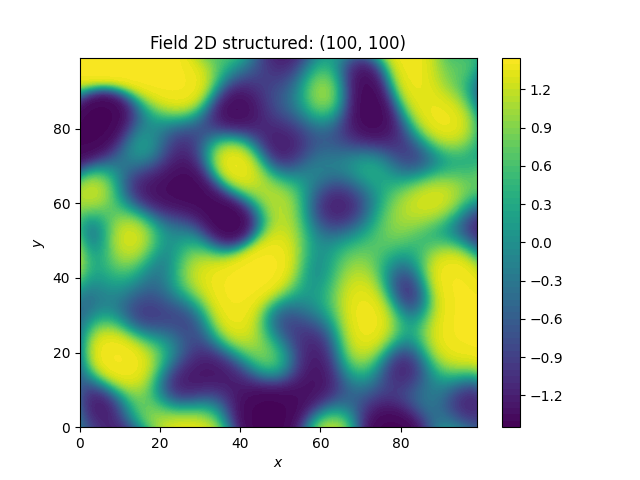# bimodal fields¶

We provide two transformations to obtain bimodal distributions:

Both transformations will preserve the mean and variance of the given field by default.```import gstools as gs

# structured field with a size of 100x100 and a grid-size of 1x1
x = y = range(100)
model = gs.Gaussian(dim=2, var=1, len_scale=10)
srf = gs.SRF(model, seed=20170519)
field = srf.structured([x, y])
gs.transform.normal_to_arcsin(srf)
srf.plot()
```

Total running time of the script: ( 0 minutes 1.044 seconds)

Gallery generated by Sphinx-Gallery